Courses

# Legendre Special Function (Part - 7)- Mathematical Methods of Physics, UGC - NET Physics Physics Notes | EduRev

## Physics for IIT JAM, UGC - NET, CSIR NET

Created by: Akhilesh Thakur

## Physics : Legendre Special Function (Part - 7)- Mathematical Methods of Physics, UGC - NET Physics Physics Notes | EduRev

The document Legendre Special Function (Part - 7)- Mathematical Methods of Physics, UGC - NET Physics Physics Notes | EduRev is a part of the Physics Course Physics for IIT JAM, UGC - NET, CSIR NET.
All you need of Physics at this link: Physics

INTEGRALS OF PRODUCTS OF THREE SPHERICAL
HARMONICS

Frequently in quantum mechanics we encounter integrals of the general form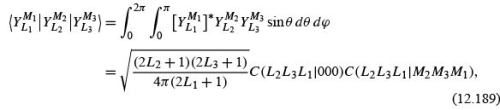in which all spherical harmonics depend on θ, ϕ . The ﬁrst factor in the integrand may come from the wave function of a ﬁnal state and the third factor from an initial state, whereas the middle factor may represent an operator that is being evaluated or whose “matrix element” is being determined.

By using group theoretical methods, as in the quantum theory of angular momentum, we may give a general expression for the forms listed. The analysis involves the vector– addition or Clebsch–Gordan coefﬁcients from Section 4.4, which are tabulated. Three general restrictions appear.

1. The integral vanishes unless the triangle condition of the L’s (angular momentum) is zero, |L1 − L3|≤ L2 ≤ L1 + L3 .

2. The integral vanishes unless M2 + M3 = M1 . Here we have the theoretical foundation of the vector model of atomic spectroscopy.

3. Finally, the integral vanishes unless the product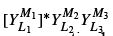is even, that is, unless L1 + L2 + L3 is an even integer. This is a parity conservation law.

The key to the determination of the integral in Eq. (12.189) is the expansion of the product of two spherical harmonics depending on the same angles (in contrast to the addition theorem), which are coupled by Clebsch–Gordan coefﬁcients to angular momentum L, M , which, from its rotational transformation properties, must be proportional to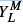(θ , ϕ ); that is,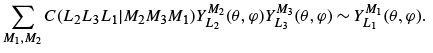Let us outline some of the steps of this general and powerful approach .
The Wigner–Eckart theorem applied to the matrix element in Eq. (12.189) yields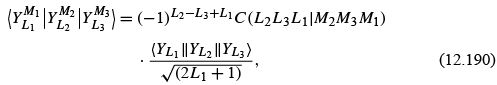where the double bars denote the reduced matrix element, which no longer depends on the M. Selection rules (1) and (2) mentioned earlier follow directly from the Clebsch– Gordan coefﬁcient in Eq. (12.190). Next we use Eq. (12.190) for M= M2 = M3 = 0in conjunction with Eq. (12.153) for m = 0, which yields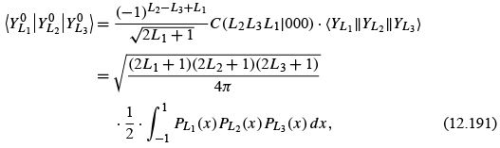where x = cos θ . By elementary methods it can be shown that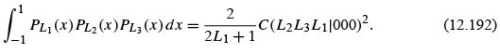Substituting Eq. (12.192) into (12.191) we obtain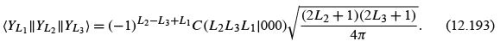The aforementioned parity selection rule (3) above follows from Eq. (12.193) in conjunction with the phase relation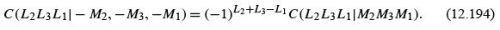Note that the vector-addition coefﬁcients are developed in terms of the Condon–Shortley phase convention,23 in which the (−1)m of Eq. (12.153) is associated with the positive m.
It is possible to evaluate many of the commonly encountered integrals of this form with the techniques already developed. The integration over azimuth may be carried out by inspection: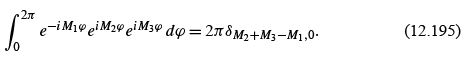Physically this corresponds to the conservation of the z component of angular momentum.

Application of Recurrence Relations

A glance at Table 12.3 will show that the θ -dependence of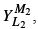, that is,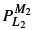(θ ), can be expressed in terms of cos θ and sin θ . However, a factor of cos θ or sin θ may be combined with the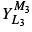factor by using the associated Legendre polynomial recurrence relations. For

instance, from Eqs. (12.92) and (12.93) we get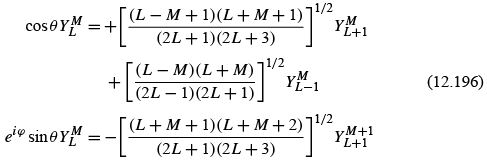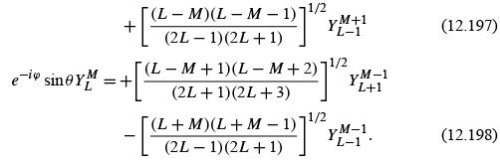Using these equations, we obtain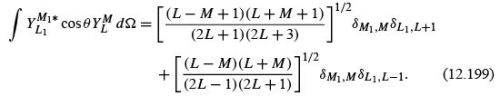The occurrence of the Kronecker delta (L1 ,L ± 1) is an aspect of the conservation of angular momentum. Physically, this integral arises in a consideration of ordinary atomic electromagnetic radiation (electric dipole). It leads to the familiar selection rule that transitions to an atomic level with orbital angular momentum quantum number L1 can originate only from atomic levels with quantum numbers L1 − 1or L1 + 1. The application to expressions such as

quadrupole moment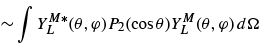is more involved but perfectly straightforward.

LEGENDRE FUNCTIONS OF THE SECOND KIND

In all the analysis so far in this chapter we have been dealing with one solution of Legendre’s equation, the solution Pn (cos θ), which is regular (ﬁnite) at the two singular points of the differential equation, cos θ =±1. From the general theory of differential equations it is known that a second solution exists. We develop this second solution, Qn , with nonnegative integer n (because Qn in applications will occur in conjunction with Pn ), byaseries solution of Legendre’s equation. Later a closed form will be obtained.

Series Solutions of Legendre’s Equation

To solve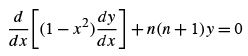(12.200)

we proceed as in Chapter 9, letting24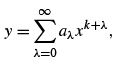(12.201)

with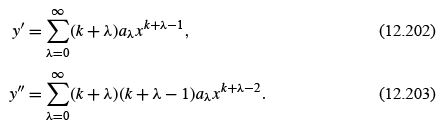Substitution into the original differential equation gives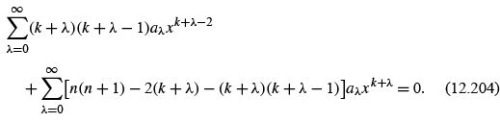The indicial equation is

k(k − 1) = 0,                        (12.205)

with solutions k = 0, 1. We try ﬁrst k = 0 with a= 1,a= 0. Then our series is described by the recurrence relation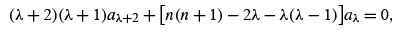(12.206)

which becomes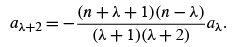(12.207)

Labeling this series, from Eq. (12.201), y(x) = pn (x ),wehave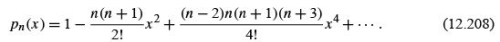The second solution of the indicial equation, k = 1, with a0 = 0,a1 = 1, leads to the recurrence relation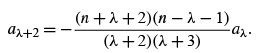(12.209)

Labeling this series, from Eq. (12.201), y(x) = qn (x ), we obtain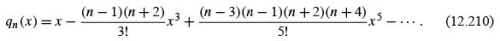Our general solution of Eq. (12.200), then, is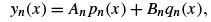(12.211)

provided we have convergence. we do not have convergence at x =±1. To get out of this difﬁculty, we set the separation constant n equal to an integer (Exercise 9.5.5) and convert the inﬁnite series into a polynomial.
For n a positive even integer (or zero), series pn terminates, and with a proper choice of a normalizing factor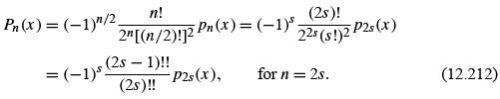If n is a positive odd integer, series qn terminates after a ﬁnite number of terms, and we write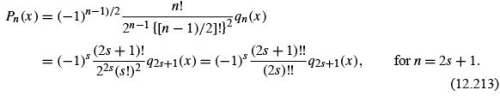Note that these expressions hold for all real values of x, −∞ <x < ∞, and for complex values in the ﬁnite complex plane. The constants that multiply pn and qn are chosen to make Pn agree with Legendre polynomials given by the generating function.
Equations (12.208) and (12.210) may still be used with n = ν , not an integer, but now the series no longer terminates, and the range of convergence becomes −1 <x < 1. The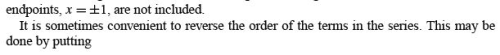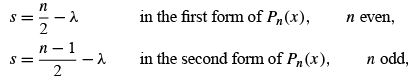so that Eqs. (12.212) and (12.213) become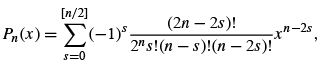(12.214)

where the upper limit s = n/2 (for n even) or (n − 1)/2(for n odd). This reproduces Eq. (12.8), which is obtained directly from the generating function. This agreement with Eq. (12.8) is the reason for the particular choice of normalization in Eqs. (12.212) and (12.213).

159 docs

,

,

,

,

,

,

,

,

,

,

,

,

,

,

,

,

,

,

,

,

,

,

,

,

;